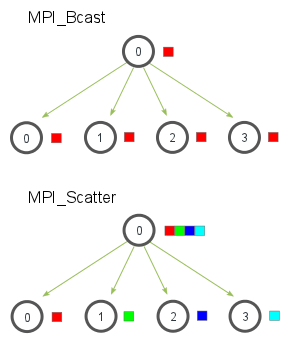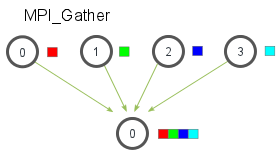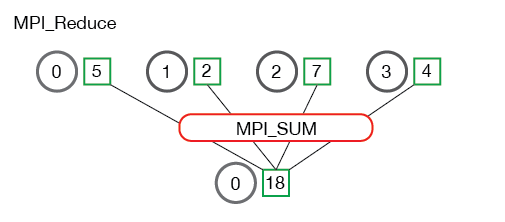## mpi4py的安装

``source activate geo_scipy``

（或者您可以从 Anaconda 应用程序启动它）

``conda install mpi4py``

## 什么是 mpi4py？

MPI for Python 为 Python 语言提供 MPI 绑定，允许程序员利用多处理器计算系统。mpi4py 是在 MPI-1/2 规范之上构建的，并提供了一个紧密遵循 MPI-2 C++ 绑定的面向对象的接口。

## mpi4py 的文档

mpi4py 的文档可以在这里找到： https ://mpi4py.scipy.org/

MPI Documents

MPI 文档仅涵盖 C 和 Fortran 实现，但 Python 语法的扩展很简单，并且在大多数情况下比等效的 C 或 Fortran 语句要简单得多。

https://mpi4py.scipy.org/docs/apiref/mpi4py.MPI-module.html

https://mpi4py.scipy.org/docs/apiref/mpi4py.MPI.Comm-class.html

## 使用 MPI 运行 Python 脚本

``\$ which mpirun/anaconda/envs/geo_scipy/bin/mpirun``

``mpirun -n 4 python script.py``

## 沟通者和等级

``from mpi4py import MPI comm = MPI.COMM_WORLDrank = comm.Get_rank()print('My rank is ',rank)``

``mpirun -n 4 python comm.py``

## 点对点通信

``from mpi4py import MPIimport numpy comm = MPI.COMM_WORLDrank = comm.Get_rank() if rank == 0:    data = {'a': 7, 'b': 3.14}    comm.send(data, dest=1)elif rank == 1:    data = comm.recv(source=0)    print('On process 1, data is ',data)``

``from mpi4py import MPIimport numpy comm = MPI.COMM_WORLDrank = comm.Get_rank() if rank == 0:    idata = 1    comm.send(idata, dest=1)elif rank == 1:    idata = comm.recv(source=0)    print('On process 1, data is ',idata)``

``from mpi4py import MPIimport numpy as np comm = MPI.COMM_WORLDrank = comm.Get_rank() if rank == 0:    # in real code, this section might    # read in data parameters from a file    numData = 10      comm.send(numData, dest=1)     data = np.linspace(0.0,3.14,numData)      comm.Send(data, dest=1) elif rank == 1:     numData = comm.recv(source=0)    print('Number of data to receive: ',numData)     data = np.empty(numData, dtype='d')  # allocate space to receive the array    comm.Recv(data, source=0)     print('data received: ',data)``

## 集体沟通

### 广播：

``from mpi4py import MPI comm = MPI.COMM_WORLDrank = comm.Get_rank() if rank == 0:    data = {'key1' : [1,2, 3],            'key2' : ( 'abc', 'xyz')}else:    data = None data = comm.bcast(data, root=0)print('Rank: ',rank,', data: ' ,data)``

``from mpi4py import MPIimport numpy as np comm = MPI.COMM_WORLDrank = comm.Get_rank() if rank == 0:    # create a data array on process 0    # in real code, this section might    # read in data parameters from a file    numData = 10      data = np.linspace(0.0,3.14,numData)  else:    numData = None # broadcast numData and allocate array on other ranks:numData = comm.bcast(numData, root=0)if rank != 0:        data = np.empty(numData, dtype='d')   comm.Bcast(data, root=0) # broadcast the array from rank 0 to all others print('Rank: ',rank, ', data received: ',data)``

### 散射：

Scatter 获取一个数组并将其连续部分分布在通信器的行列中。这是来自 http://mpitutorial.com 的图片，它说明了广播和分散之间的区别：``from mpi4py import MPIimport numpy as np comm = MPI.COMM_WORLDsize = comm.Get_size() # new: gives number of ranks in commrank = comm.Get_rank() numDataPerRank = 10  data = Noneif rank == 0:    data = np.linspace(1,size*numDataPerRank,numDataPerRank*size)    # when size=4 (using -n 4), data = [1.0:40.0] recvbuf = np.empty(numDataPerRank, dtype='d') # allocate space for recvbufcomm.Scatter(data, recvbuf, root=0) print('Rank: ',rank, ', recvbuf received: ',recvbuf)``

## 搜集：``from mpi4py import MPIimport numpy as np comm = MPI.COMM_WORLDsize = comm.Get_size()rank = comm.Get_rank()    numDataPerRank = 10  sendbuf = np.linspace(rank*numDataPerRank+1,(rank+1)*numDataPerRank,numDataPerRank)print('Rank: ',rank, ', sendbuf: ',sendbuf) recvbuf = Noneif rank == 0:    recvbuf = np.empty(numDataPerRank*size, dtype='d')   comm.Gather(sendbuf, recvbuf, root=0) if rank == 0:    print('Rank: ',rank, ', recvbuf received: ',recvbuf)``

## 减少：

MP`reduce`操作从每个进程的数组中获取值，并将它们简化为根进程上的单个结果。这本质上就像从每个进程向根进程发送一个有点复杂的命令，然后让根进程执行归约操作。值得庆幸的是，MPI`reduce` 用一个简洁的命令完成了所有这些工作。

~~~python comm.Reduce(send_data, recv_data, op=, root=0) ~~~哪里`send_data`是通信器上所有进程发送的数据，`recv_data`是根进程上将接收所有数据的数组。请注意，发送和接收数组具有相同的大小。里面的所有数据副本 `send_data`都会按照指定的减少`<operation>`。一些常用的操作是： – MPI_SUM – 对元素求和。- MPI_PROD – 将所有元素相乘。- MPI_MAX – 返回最大元素。- MPI_MIN – 返回最小元素。``from mpi4py import MPIimport numpy as np comm = MPI.COMM_WORLDrank = comm.Get_rank() # Create some np arrays on each process:# For this demo, the arrays have only one# entry that is assigned to be the rank of the processorvalue = np.array(rank,'d') print(' Rank: ',rank, ' value = ', value) # initialize the np arrays that will store the results:value_sum      = np.array(0.0,'d')value_max      = np.array(0.0,'d') # perform the reductions:comm.Reduce(value, value_sum, op=MPI.SUM, root=0)comm.Reduce(value, value_max, op=MPI.MAX, root=0) if rank == 0:    print(' Rank 0: value_sum =    ',value_sum)    print(' Rank 0: value_max =    ',value_max)``